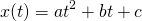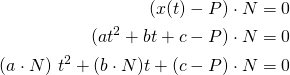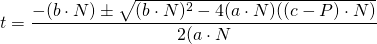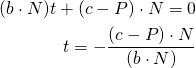# Modeling a projectile's motion

Thanks for letting me know. I never use dark theme on the dev forum so I would have never known. Should be fixed now via block quotes.

8 Likes
8 Likes

Wonderful tutorial! This will finally allow me to make a few throwing systems I’ve been thinking of.

4 Likes

That’s about the best I can do unfortunately15 Likes

This is amazing! I’m so excited to finally learn how to do this. Thanks!!

3 Likes

I uploaded the Tool to Roblox Library and a .rbmx file here for ease of accessibility.

Projectile Script.rbxm (4.4 KB)

@EgoMoose Quick question what is t and nt?

Those Variables aren’t self explanatory, they don’t make sense.

11 Likes

`t` is time it takes to reach the target. `nt` is the elapsed time.

7 Likes

So recently @Maximum_ADHD posted this on twitter and through a series of events I was asked if I would post a tutorial showing how to do it. I’m not sure if this is exactly how Clone did it, but this is how I approached it.

So to start off let’s cover the process that doing something like this would entail.

1. Start with a position and velocity which we can use the above equations to find the projectile’s path.
2. Find where this path intersects with in game geometry.
3. Reflect the current velocity against the geometry’s surface.
4. Use the intersection position and reflected velocity to repeat from step 1 as many times as desired.

We already know how to do steps 1 and 4 from the above post so all we need to cover are steps 2 and 3.

To find where our projectile intersects with a surface we’ll do two things.

We’ll first split our projectile’s path into multiple rays which can then be used to find an intersection with in game geometry.

``````local g = Vector3.new(0, game.Workspace.Gravity, 0)

local function x(t, v0, x0)
return 0.5*g*t*t + v0*t + x0
end

local function intersection(x0, v0)
local t = 0
local hit, pos, normal

repeat
local p0 = x(t, v0, x0)
local p1 = x(t + 0.1, v0, x0)
t = t + 0.1

local ray = Ray.new(p0, p1 - p0)
hit, pos, normal = game.Workspace:FindPartOnRay(ray)
until (hit or t > 5)

-- so our plane is defined by the pos and normal variables
end
``````

This will provide two key pieces of information; an estimated intersection position and a surface normal. We’ll then use those pieces of information to do a plane-quadratic intersection which will give us an exact intersection value.

Using a general form of the quadratic formula:We know the quadratic will intersect with a plane defined by `P` and `N` when:This gives us two situations where we solve for t differently.

The first being when `a . N ≠ 0` in which case we use the quadratic formula.The second being when `a . N = 0` in which case we simple solve the linear equation for t:In code this translates to:

``````local function planeQuadraticIntersection(v0, x0, p, n)
local a = (0.5*g):Dot(n)
local b = v0:Dot(n)
local c = (x0 - p):Dot(n)

if (a ~= 0) then
local d = math.sqrt(b*b - 4*a*c)
return (-b - d)/(2*a)
else
return -c / b
end
end
``````

All that’s left is to solve step 3 which is to reflect our velocity vector against the surface normal. This is simple enough and is currently explained on the dot product devhub wiki page. The only thing you might do here is slightly damp the velocity such that your reflection loses some energy as it bounces.

When you put all that together you get:

It’s not perfect mainly because how velocity is damped, but it’s a pretty good projection of the path traveled. Enjoy!

Trajectory.rbxl (25.2 KB)

118 Likes

PLS, can you put the formulas to block quote, bec this is unreadeable, at topic, it isnt best, but it is much better.

4 Likes

A very well done tutorial with a great explanation with some beautiful representations. However It is harder for younger developers like me to understand all this hard math that contains integrals and derives, I tried learning and studying them on my own, I also had been discussing with teachers about how I could learn them. But the problem still remains. I would highly appreciate if you could do another post where you explain everything with some simple math without any hard calculus. I really regret that I am not able to learn and understand everything you mentioned. Amazing tutorial and keep up the great work.

9 Likes

Is it possible to do this with a bodyvelocity?

5 Likes

Heya, sorry to necro-bump this but i was wondering how i could check when the :Travel() method is finished or atleast approximate how long it might take.

Sorry again for bumping

2 Likes

Does the part’s mass matter in this equation, Also I’m trying to do this with a body force in the part that counteracts gravity not sure how to incorporate that with the gravity calculation.

3 Likes

How would we go about keeping a consistant height on the trajectory? When you point the mouse far the launch looks a bit crazy fir most cases like throwing a basketball or launching out of a cannon.

4 Likes

Mass should not matter. Acceleration due to gravity remains the same for all objects.

8 Likes

Never knew this was possible! I used to simulate it in a for loop then just put bricks at its positions

2 Likes

This topic can use integral calc, but it mostly regards with physics and projectile motion.
A short 13 minute video to help you understand a bit about projectile motion is rather simple in the way the video explains it. I’m obviously not going to type a whole page about projectile motion when there’s a video that does it all.
https://youtu.be/M8xCj2VPHas
This is for projectile motion, and you’ll immediately notice it’s very similar to the tutorial. In the equations, they just use different variables and the terms are in different order, but I’m sure you can easily understand the equations.

9 Likes

Love the tutorial, especially showing the importance of math to doing this stuff!

One question though, if I don’t have a sphere following the path but a rod, what would be the best way to rotate it so that it is always pointing in the direction of the arc? At first I was thinking about doing a BodyAngularVelocity from 0 to pi, but that won’t work in a local script. Would I have to use CFrames then?

EDIT: Ok, here’s what I’ve got so far:
Right before the `while (nt < t*2)` loop, I put this in to set the starting angle of the projectile and point it at the target.

``````local angle = math.pi / 4
p.CFrame = CFrame.new(p.Position, hrp.Position)``````

Then, inside of the while loop I have:

``p.CFrame = p.CFrame * CFrame.Angles(0, angle, 0)``

And after the RenderStepped update:

``angle = (math.pi / 4) - ((nt / (t * 2)) * (math.pi / 2))``

What this should do is start the projectile pointing at the target, then up at a 45 degree angle. Then, on each render step, it subtracts from that angle a proportion of the full rotation of the projectile. The end result is moving from a +45 angle to a -45 angle by the end of the animation.

However, when I run it, I don’t see the projectile actually rotating. I printed out all my angles as it calculates them, and they look correct, running from +.76 to -.80.

Can anyone see what I’m doing wrong?

EDIT 2: I figured out some of the mistakes I made in that code. Turns out that I can’t do the CFrame.Angles with the argument of the angle I want it pointed to, but I have to use an argument for how much I want it rotated by.

So instead of calculating the necessary angle for each step, I just have to calculate the change in angle between each frame:

``local angleDelta = ((math.pi / 4) / t) / 60``

Because I couldn’t see a good way to calculate the number of times the animation loop will run, I took the amount of the rotation (45 degrees) divided by the time to take that rotation (t) divided by the number of frames per second (I assumed 60 because that is the cap and I don’t know a good way to get the actual value).

Then, in the animation loop, I have:

``projectile.CFrame = projectile.CFrame * CFrame.Angles(-(angleDelta), 0, 0)``

Now when I have a static projectile that is not moving, it rotates the correct amount in the correct duration. But when I try to combine it with the CFrame change from `projectile.CFrame = CFrame.new((.5 * g * nt * nt) + (v0 * nt) + source.Position)`, it doesn’t rotate. I also tried combining the two together with a multiplication, but that doesn’t work either.

Any ideas why I can’t get the two working together?

5 Likes

Sorry I for whatever reason didn’t see this question when you originally posted it.

For this I would use the velocity equation to get the direction you’re object is going to be facing and then from there you can use `CFrame.lookAt` or `CFrame.fromMatrix`.

``````local g, v0, x0 -- define these

local function x(t)
return 0.5*g*t*t + v0*t + x0
end

local function v(t)
return g*t + v0
end

local t = 0
game:GetService("RunService").Heartbeat:Connect(function(dt)
t = t + dt
local position = x(t)
local velocity = v(t)

local cframe = CFrame.lookAt(position, position + velocity)
end)
``````

Now the one issue you may find with this is when the parabola reaches its peak then velocity will have a magnitude of zero (as shown when deriving the max jump height in the OP).

In that case we need to handle the situation a little differently:

``````local t = 0
game:GetService("RunService").Heartbeat:Connect(function(dt)
t = t + dt
local position = x(t)
local velocity = v(t)

if velocity:Dot(velocity) == 0 then -- magnitude^2 == 0
velocity = v(t - dt) * Vector3.new(1, 0, 1)
end

local cframe = CFrame.lookAt(position, position + velocity)
end)
``````

Hope that helps!

28 Likes

This is exactly what I was looking for! Thank you so much1 Like